d & f – block – JEE Main Previous Year Questions with Solutions
JEE Main Previous Year Papers Questions of Chemistry with Solutions are available at eSaral. Practicing JEE Main chapter wise questions of Chemistry will help the JEE aspirants in realizing the question pattern as well as help in analyzing weak & strong areas.SimulatorPrevious Years AIEEE/JEE Main Question
Q. In context with the transition elements, which of the following statements is incorrect?(1) In the highest oxidation states of the first five transition elements (Sc to Mn), all the 4sand 3d electrons are used for bonding.(2) Once the d5 configuration is exceeded, the tendency to involve all the 3d electrons inbonding decreases.(3) In addition to the normal oxidation states, the zero oxidation state is also shown by theseelements in complexes.(4) In the highest oxidation states, the transition metal show basic character and form cationiccomplexes. [AIEEE-2009]

Download eSaral App for Video Lectures, Complete Revision, Study Material and much more...

Sol. (4)

Q. Iron exhibits +2 and +3 oxidation states. Which of the following statements about iron is incorrect ?(1) Ferrous compounds are more easily hydrolysed than the corresponding ferric compounds.(2) Ferrous oxide is more basic in nature than the ferric oxide.(3) Ferrous compounds are relatively more ionic than the corresponding ferric compounds.(4) Ferrous compounds are less volatile than the corresponding ferric compounds. [AIEEE-2012]

Download eSaral App for Video Lectures, Complete Revision, Study Material and much more...

Sol. (1)

Q. Consider the following reaction : $\mathrm{xMnO}_{4}^{-}+\mathrm{yC}_{2} \mathrm{O}_{4}^{2-}+\mathrm{zH}^{+} \rightarrow \mathrm{xMn}^{2+}+2 \mathrm{yCO}_{2}+\frac{\mathrm{Z}}{2} \mathrm{H}_{2} \mathrm{O}$The values of x, y and z in the reaction are respectively :-(1) 5,2 and 16(2) 2,5 and 8(3) 2, 5 and 16(4) 5,2 and 8 [JEE MAIN-2013]

Download eSaral App for Video Lectures, Complete Revision, Study Material and much more...

Sol. (3)

Q. Which of the following arrangements does not represent the correct order of the property stated against it ?(1) $\mathrm{V}^{2+}<\mathrm{Cr}^{2+}<\mathrm{Mn}^{2}<\mathrm{Fe}^{2+}$: paramagnetic behaviour(2) $\mathrm{Ni}^{2+}<\mathrm{Co}^{2+}<\mathrm{Fe}^{2+}<\mathrm{Mn}^{2+}$: ionic size(3)$\mathrm{Co}^{3+}<\mathrm{Fe}^{3+}<\mathrm{Cr}^{3+}<\mathrm{Sc}^{3+}$: stability in aqueous solution(4) $\mathrm{Sc}<\mathrm{Ti}<\mathrm{Cr}<$Mn : number of oxidation states [JEE MAIN-2013]

Download eSaral App for Video Lectures, Complete Revision, Study Material and much more...

Sol. (1)

Q. Potassium dichromate when heated with concentrated sulphuric acid and a soluble chloride, gives brown – red vapours of:(1) $\mathrm{CrO}_{3}$(2) $\mathrm{Cr}_{2} \mathrm{O}_{3}$(3) $\mathrm{CrCl}_{3}$(4) $\mathrm{CrO}_{2} \mathrm{Cl}_{2}$ [JEE MAIN-2013, Online]

Download eSaral App for Video Lectures, Complete Revision, Study Material and much more...

Sol. (4)Explanation. $\mathrm{K}_{2} \mathrm{Cr}_{2} \mathrm{O}_{7}+6 \mathrm{H}_{2} \mathrm{SO}_{4}+4 \mathrm{NaCl} \longrightarrow 2 \mathrm{KHSO}_{4}+4 \mathrm{NaHSO}_{4}+2 \mathrm{CrO}_{2} \mathrm{Cl}_{2}+3 \mathrm{H}_{2} \mathrm{O}$

Q. The element with which of the following outer electron configuration may exhibit the largest number of oxidation states in its compounds :(1) $3 \mathrm{d}^{7} 4 \mathrm{s}^{2}$(2) $3 \mathrm{d}^{8} 4 \mathrm{s}^{2}$( 3) (3) $3 \mathrm{d}^{5} 4 \mathrm{s}^{2}$(4) (4) $3 \mathrm{d}^{6} 4 \mathrm{S}^{2}$ [JEE MAIN-2013, Online]

Download eSaral App for Video Lectures, Complete Revision, Study Material and much more...

Sol. (3)Explanation$\mathrm{Mn} \longrightarrow \mathrm{Mn}^{+7}$

Q. When a small amount of $\mathrm{KMnO}_{4}$ is added to concentrated $\mathrm{H}_{2} \mathrm{SO}_{4}$a green oily compound is obtained which is highly explosive in nature. Compound may be:(1) $\mathrm{Mn}_{2} \mathrm{O}_{3}$(2) MnSO $_{4}$(3) $\mathrm{Mn}_{2} \mathrm{O}_{7}$( 4) $\mathrm{MnO}_{2}$ [JEE MAIN-2013, Online]

Download eSaral App for Video Lectures, Complete Revision, Study Material and much more...

Sol. (3)ExplanationQ. Which series of reactions correctly represents chemical relations related to iron and its compound?[JEE MAIN-2014]

Download eSaral App for Video Lectures, Complete Revision, Study Material and much more...

Sol. (2)

Q. The equation which is balanced and represents the correct product (s) is:[JEE MAIN-2014]

Download eSaral App for Video Lectures, Complete Revision, Study Material and much more...

Sol. (4)

Q. Which of the following is not formed when $\mathrm{H}_{2} \mathrm{S}$ reacts with acidic $\mathrm{K}_{2} \mathrm{Cr}_{2} \mathrm{O}_{7}$solution ?( 1) $\mathrm{K}_{2} \mathrm{SO}_{4}$(2) $\mathrm{Cr}_{2}\left(\mathrm{SO}_{4}\right)_{3}$(3) $\mathrm{S}$(4) $\mathrm{CrSO}_{4}$ [JEE MAIN-2014, Online]

Download eSaral App for Video Lectures, Complete Revision, Study Material and much more...

Sol. (4)

Q. Copper becomes green when exposed to moist air for a long period. This is due to :-(1) the formation of a layer of cupric oxide on the surface of copper.(2) the formation of basic copper sulphate layer on the surface of the metal(3) the formation of a layer of cupric hydroxide on the surface of copper.(4) the formation of a layer of basic carbonate of copper on the surface of copper. [JEE MAIN-2014, Online]

Download eSaral App for Video Lectures, Complete Revision, Study Material and much more...

Sol. (4)

Q. Which one of the following exhibits the largest number of oxidation states ?(1) Mn(25)(2) V(23)(3) Cr (24)(4) Ti (22) [JEE MAIN-2014, Online]

Download eSaral App for Video Lectures, Complete Revision, Study Material and much more...

Sol. (1)

Q. How many electrons are involved in the following redox reaction?$\mathrm{Cr}_{2} \mathrm{O}_{7}^{2-}+\mathrm{Fe}^{2+}+\mathrm{C}_{2} \mathrm{O}_{4}^{2-} \rightarrow \mathrm{Cr}^{3+}+\mathrm{Fe}^{3+}+\mathrm{CO}_{2}$ (Unbalanced)(1) 3(2) 4(3) 5(4) 6 [JEE MAINS-2014,Online]

Download eSaral App for Video Lectures, Complete Revision, Study Material and much more...

Sol. (4)

Q. Amongst the following, identify the species with an atom in +6 oxidation state:(1) $\left[\mathrm{MnO}_{4}\right]^{-}$(2) $\left[\mathrm{Cr}(\mathrm{CN})_{6}\right]^{3-}$(3) $\mathrm{Cr}_{2} \mathrm{O}_{3}$(4) $\mathrm{CrO}_{2} \mathrm{Cl}_{2}$ [JEE MAIN-2014, Online]

Download eSaral App for Video Lectures, Complete Revision, Study Material and much more...

Sol. (4)

Q. Match the catalysts to the correct processes :-(1) A-ii, B-iii, C-iv, D-i(2) A-iii, B-i, C-ii, D-iv(3) A-iii, B-ii, C-iv, D-i(4) A-ii, B-i, C-iv, D-iii [JEE MAIN-2015]

Download eSaral App for Video Lectures, Complete Revision, Study Material and much more...

Sol. (4)

Q. Which of the following statements is false :-(1) has a Cr – O – Cr bond(2) is tetrahedral in shape(3) $\mathrm{Na}_{2} \mathrm{Cr}_{2} \mathrm{O}_{7}$ is a primary standard in volumetry(4) $\mathrm{Na}_{2} \mathrm{Cr}_{2} \mathrm{O}_{7}$ is less soluble than $\mathrm{K}_{2} \mathrm{Cr}_{2} \mathrm{O}_{7}$ [JEE MAIN-2015, Online]

Download eSaral App for Video Lectures, Complete Revision, Study Material and much more...

Sol. (3)

Polymer – JEE Main Previous Year Questions with Solutions
JEE Main Previous Year Papers Questions of Chemistry with Solutions are available at eSaral. Practicing JEE Main chapter wise questions of Chemistry will help the JEE aspirants in realizing the question pattern as well as help in analyzing weak & strong areas.  Simulator Previous Years AIEEE/JEE Main Questions
Q. Buna–N synthetic rubber is a copolymer of :-[AIEEE-2009]

Download eSaral App for Video Lectures, Complete Revision, Study Material and much more...

Sol. (1)Buna-N is actually abbrebiated form, where Bu represents 1, 3-butadiene, Na representssodium and N represents nitrile (acrylonitrile).Thus, Buna-N is a copolymer of 1, 3-butadiene and acrylonitrile usually copolymer of1, 3-butadiene, and acrylonitrile usually ploymerise in the presence of sodium.

Q. The polymer containing strong intermolecular forces e.g. hydrogen bonding, is :-(1) natural rubber(2) teflon(3) nylon 6, 6(4) polystyrene [AIEEE-2010]

Download eSaral App for Video Lectures, Complete Revision, Study Material and much more...

Sol. (3)

Q. Thermosetting polymer, Bakelite is formed by the reaction of phenol with :-(1) $\mathrm{CH}_{3} \mathrm{CH}_{2} \mathrm{CHO}$(2) $\mathrm{CH}_{3} \mathrm{CHO}$(3) HCHO(4) HCOOH [AIEEE-2011]

Download eSaral App for Video Lectures, Complete Revision, Study Material and much more...

Sol. (3)Bakelite is a thermosetting polymer formed by the condensation reaction of phenol withHCHO in the presense of conc. $\mathrm{H}_{2} \mathrm{SO}_{4}$It is thus, a cross-linked polymer in which condesation takes place at O- and p- positionsSo HCHO is the corerect option.

Q. The species which can best serve as an initiator for the cationic polymerization is :-(1) BuLi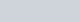(3) HNO $_{3}$(4) $\mathrm{AlCl}_{3}$ [AIEEE-2012]

Download eSaral App for Video Lectures, Complete Revision, Study Material and much more...

Sol. (4)Electron – deficient species (Lawis acid) like $\mathrm{AlCl}_{3}, \mathrm{BF}_{3}$ etc.are is used as initiator for cationic polymerisation.

Q. Which one is classified as a condensation polymer?(1) Teflon(2) Acrylonitrile(3) Dacron(4) Neoprene [JEE-Main 2014]

Download eSaral App for Video Lectures, Complete Revision, Study Material and much more...

Sol. (3)Q. Which one of the following structures represents the neoprene polymer :-[JEE-Main(Online) – 2015]

Download eSaral App for Video Lectures, Complete Revision, Study Material and much more...

Sol. (3)Q. Which polymer is used in the manufacture of paints and lacquers ?(1) Polypropene(2) Poly vinyl chloride(3) Bakelite(4) Glyptal [JEE- Main – 2015]

Download eSaral App for Video Lectures, Complete Revision, Study Material and much more...

Sol. (4)Q. Which of the following statements about low density polythene is FALSE ?(1) It is used in the manufacture of buckets, dust-bins etc.(2) Its synthesis requires high pressure(3) It is a poor conductor of electricity(4) Its synthesis requires dioxygen or a peroxide initiator as a catalyst. [JEE Main 2016]

Download eSaral App for Video Lectures, Complete Revision, Study Material and much more...

Sol. (1)Low density polythene : It is obtained by the polymerisation of ethene under high presure of 1000-2000 atm. at a temp. of 350 K to 570 K in the pressure of traces of dioxygen or a peroxide

Q. Assertion : Rayon is a semisynthetic polymer whose properties are better thannatural cotton.Reason : Mechanical and aesthetic properties of cellulose can be improved by acetylation.(1) Both assertion and reason are incorrect(2) Assertion is incorrect statement, but the reason is correct(3) Both assertion and reason are correct, but the reason is not the correct explanation for theassertion(4) Both assertion and reason are correct, and the reason is the correct explanation for the assertion [JEE Main (Online) 2016]

Download eSaral App for Video Lectures, Complete Revision, Study Material and much more...

Sol. (4)

Q. Which of the following polymers is synthesized using a free radical polymerizationtechnique ? (1) Terylene(2) Teflon(3) Nylon 6,6(4) Melamine polymer [JEE Main (Online) 2016]

Download eSaral App for Video Lectures, Complete Revision, Study Material and much more...

Sol. (2)[esquestion] The formation of which of the following polymers involves hydrolysis reaction ?(1) Nylon 6(2) Bakelite(3) Nylon 6, 6(4) Terylene#tag# [JEE – Main 2017]

Chemistry in Everyday Life – JEE Main Previous Year Questions with Solutions
JEE Main Previous Year Papers Questions of Chemistry with Solutions are available at eSaral. Practicing JEE Mains chapter wise questions of Chemistry will help the JEE aspirants in realizing the question pattern as well as help in analyzing weak & strong areas.SimulatorPrevious Years AIEEE/JEE Mains Questions
Q. Which artificial sweetener contains chlorine ?(1) Aspartame(2) Saccharin(3) Sucralose(4) Alitame [JEE-Main(Online) – 2015]

Download eSaral App for Video Lectures, Complete Revision, Study Material and much more...

Sol. (3)Q. Thiol group is present in :-(1)Methionine(2) Cytosine(3) Cystine(4) Cysteine [JEE-Main-2016]

Download eSaral App for Video Lectures, Complete Revision, Study Material and much more...

Sol. (4)Among 20 naturally occuring amino acids “Cysteine” has ‘– SH’ or thiol functional group.Q. The distillation technique most suited for separating glycerol from spent-lye in the soap industry is:(1) Distillation under reduced pressure(2) Simple distillation(3) Fractional distillation(4) Steam distillation (Jee-Main-2016)

Download eSaral App for Video Lectures, Complete Revision, Study Material and much more...

Sol. (1)(1) Distillation under reduced pressure.Glycerol $\left(\mathrm{B} . \mathrm{P} .290^{\circ} \mathrm{C}\right)$ is separated from spent lye in the soap industry by distillation under reduced pressure, as for simple distillation very high temperature is required which might decompose the component.

Q. The predominant form of histamine present in human blood is $\left(\mathrm{pK}_{\mathrm{a}}\right.$, Histidine = 6.0)(1)(2)(3)(4)Download eSaral App for Video Lectures, Complete Revision, Study Material and much more...

Sol. (3)Carboxylic Acid – JEE Main Previous Year Questions with Solutions
JEE Main Previous Year Papers Questions of Chemistry with Solutions are available at eSaral. Practicing JEE Mains chapter wise questions of Chemistry will help the JEE aspirants in realizing the question pattern as well as help in analyzing weak & strong areas.SimulatorPrevious Years AIEEE/JEE Mains Questions
Q. In the chemical reactions,(1) Nitrobenzene and chlorobenzene(2) Nitrobenzene and fluorobenzene(3) Phenol and benzene(4) Benzene diazonium chloride and fluorobenzene [AIEEE-2010]

Download eSaral App for Video Lectures, Complete Revision, Study Material and much more...

Sol. (4)Q. In the chemical reactions(1) Fluorobenzene and phenol(2)Benzene diazonium chloride and benzonitrile(3) Nitrobenzene and chlorobenzene(4) Phenol and bromobenzene [AIEEE-2011]

Download eSaral App for Video Lectures, Complete Revision, Study Material and much more...

Sol. (2)Q. Compound (A), $\mathrm{C}_{8} \mathrm{H}_{9} \mathrm{Br}$, gives a white precipitate when warmed with alcoholic $\mathrm{AgNO}_{3}$. Oxidation of (A) gives an acid (B), $\mathrm{C}_{8} \mathrm{H}_{6} \mathrm{O}_{4}$. (B) easily forms anhydride on heating. Identify the compound (A):[AIEEE-2013]

Download eSaral App for Video Lectures, Complete Revision, Study Material and much more...

Sol. (4)since B on heating gives anhydride so B is 1,2 dicarboxylic acid. It contains two Benzylic carbon at 1,2 position so on oxidation it produces 1, 2-dicarboxylic acidQ. An organic compound A upon reacting with $\mathrm{NH}_{3}$ gives B. On heating, B gives C. C in presence of KOH reacts with $\mathbf{B} \mathbf{r}_{2}$ to give $\mathrm{CH}_{3} \mathrm{CH}_{2} \mathrm{NH}_{2}$. A is :-(1) $\mathrm{CH}_{3} \mathrm{COOH}$(2) $\mathrm{CH}_{3} \mathrm{CH}_{2} \mathrm{CH}_{2} \mathrm{COOH}$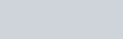(4) $\mathrm{CH}_{3} \mathrm{CH}_{2} \mathrm{COOH}$ [AIEEE-2013]

Download eSaral App for Video Lectures, Complete Revision, Study Material and much more...

Sol. (4)Q. On heating an aliphatic primary amine with chloroform & ethenolic potassium hydroside the organic compound formed is(1) An alkyl cyanide(2) An alkyl isocyanide(3) an alkanol(4) an alkanediol [AIEEE-2014]

Download eSaral App for Video Lectures, Complete Revision, Study Material and much more...

Sol. (2)It is a carbyl amine reaction used for identification of primary amine also known as isocynide test because of offensive smell of isocynide.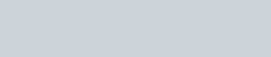Q. In the reaction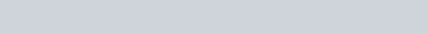(1) Ethylene(2) Acetyl chloride(3) Acetaldehyde(4) Acetylene. [AIEEE-2014]

Download eSaral App for Video Lectures, Complete Revision, Study Material and much more...

Sol. (1)Q. In the presence of a small amount of phosphorous, aliphatic carboxylic acids react with chlorine or bromine to yield a compound in which  – hydrogen has been replaced by halogen. This reaction is known as :(1) Etard reaction(2) Hell – Volhard – Zelinsky reaction(3) Wolff – Kischner reaction(4) Rosenmund reaction [JEE(Main)-2015]

Download eSaral App for Video Lectures, Complete Revision, Study Material and much more...

Sol. (2)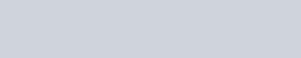Q. In the Hofmann bromamide degradation reaction, the number of moles of NaOH and $\mathrm{Br}_{2}$ used per mole of amine produced are :[JEE(Main)-2016]

Download eSaral App for Video Lectures, Complete Revision, Study Material and much more...

Sol. (1)Q. The major product obtained in the following reaction is :-[JEE – Main 2017]

Download eSaral App for Video Lectures, Complete Revision, Study Material and much more...

Sol. (1)DIBAL – H is electrophilic reducing agent reduces cynide, esters, lactone, amide, carboxylic acid into corresponding Aldehyde (partial reduction)

Q. Sodium salt of an organic acid ‘X’ produces effervescence with conc. $\mathrm{H}_{2} \mathrm{SO}_{4}$. ‘X’ reacts with the acidified aqueous CaCl2 solution to give a white precipitate which decolourises acidic solution of $\mathrm{KMnO}_{4}$. ‘X’ is :-(1) $\mathrm{C}_{6} \mathrm{H}_{5} \mathrm{COONa}$(2) HCOONa(3) CH3COONa(4) $\mathrm{Na}_{2} \mathrm{C}_{2} \mathrm{O}_{4}$ [JEE – Main – 2017]

Download eSaral App for Video Lectures, Complete Revision, Study Material and much more...

Sol. (4)Q. Which of the following compounds will be suitable for Kjeldahl’s method for nitrogen estimation ?[JEE(Main)-2018]

Download eSaral App for Video Lectures, Complete Revision, Study Material and much more...

Sol. (1)Kjeldahl’s is suitable for Aniline. This method is used for quantitative analysis of N compound in organic substance $\left(\mathrm{NH}_{3} / \mathrm{NH}_{4}^{+}\right) .$

Carbonyl Compounds – JEE Main Previous Year Questions with Solutions
JEE Main Previous Year Papers Questions of Chemistry with Solutions are available at eSaral. Practicing JEE Mains chapter wise questions of Chemistry will help the JEE aspirants in realizing the question pattern as well as help in analyzing weak & strong areas.Simulator Previous Years AIEEE/JEE Mains Questions
Q. Which of the following on heating with aqueous KOH, produces acetaldehyde ?(1) $\mathrm{CH}_{2} \mathrm{ClCH}_{2} \mathrm{Cl}$(2) $\mathrm{CH}_{3} \mathrm{CHCl}_{2}$(3) $\mathrm{CH}_{3} \mathrm{COCl}$(4) $\mathrm{CH}_{3} \mathrm{CH}_{2} \mathrm{Cl}$ [AIEEE-2009]

Download eSaral App for Video Lectures, Complete Revision, Study Material and much more...

Sol. (2)

Q. In Cannizzaro reaction given below :-$2 \mathrm{Ph} \mathrm{CHO}$ $\mathrm{PhCH}_{2}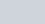\mathrm{OH}+\mathrm{PhCO}_{2}^{\Theta}$ the slowest step is :-(1) The abstraction of proton from the carboxylic group(2) The deprotonation of $\mathrm{CH}_{2} \mathrm{OH}$(3) The attack of $: \stackrel{\ominus}{\mathrm{O}} \mathrm{H}$ at the carboxyl group(4) The transfer of hydride to the carbonyl group [AIEEE-2009]

Download eSaral App for Video Lectures, Complete Revision, Study Material and much more...

Sol. (4)

Q. One mole of a symmetrical alkene on ozonolysis gives two moles of an aldehyde having a molecular mass of 44 u. The alkene is :-(1) Ethene (2) Propene (3) 1-Butene (4) 2-Butene [AIEEE-2010]

Download eSaral App for Video Lectures, Complete Revision, Study Material and much more...

Sol. (4)

Q. Ozonolysis of an organic compound gives formaldehyde as one of the products. This confirms the presence of :-(1) An isopropyl group(2) An acetylenic triple bond(3) Two ethylenic double bonds(4) A vinyl group [AIEEE-2011]

Download eSaral App for Video Lectures, Complete Revision, Study Material and much more...

Sol. (4)

Q. Ozonolysis of an organic compound ‘A’ produces acetone and propionaldehyde in equimolar mixture. Identify ‘A’ from the following compounds : –(1) 2-Methyl – 1- pentene(2) 1-Pentene(3) 2-Pentene(4) 2-Methyl-2-pentene [AIEEE-2011]

Download eSaral App for Video Lectures, Complete Revision, Study Material and much more...

Sol. (4)

Q. Trichloroacetaldehyde was subjected to Cannizzaro’s reaction by using NaOH. The mixture of the products contains sodium trichloroacetate and another compound. The other(1) 2,2,2–Trichloropropanol(2) Chloroform(3) 2,2,2–Trichloroethanol(4) Trichloromethanol [AIEEE-2011]

Download eSaral App for Video Lectures, Complete Revision, Study Material and much more...

Sol. (3)

Q. Silver Mirror test is given by which one of the following compounds?(1) Formaldehyde (2) Benzophenone (3) Acetaldehyde (4) Acetone [AIEEE-2011]

Download eSaral App for Video Lectures, Complete Revision, Study Material and much more...

Sol. (1,3)

Q. In the given transformation, which of the following is the most appropriate reagent ?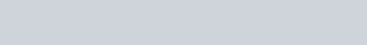(1) $\mathrm{NaBH}_{4}$(2) $\mathrm{NH}_{2} \mathrm{NH}_{2}, \stackrel{\ominus}{\mathrm{O}} \mathrm{H}$(3) Zn – Hg / HCl$(4) \mathrm{Na}, \mathrm{Liq.NH}_{3}$ [AIEEE-2012]

Download eSaral App for Video Lectures, Complete Revision, Study Material and much more...

Sol. (2)

Q. Iodoform can be prepared from all except :-(1) Isobutyl alcohol(2) Ethyl methyl ketone(3) Isopropyl alcohol(4) 3-Methyl–2–butanone [AIEEE-2012]

Download eSaral App for Video Lectures, Complete Revision, Study Material and much more...

Sol. (1)

Q. A compound with molecular mass 180 is acylated with $\mathrm{CH}_{3} \mathrm{COCl}$ to get a compound with molecular mass 390. The number of amino groups present per molecule of the former compound is :-(1) 2 (2) 5 (3) 4 (4) 6 [JEE(Main)-2013]

Download eSaral App for Video Lectures, Complete Revision, Study Material and much more...

Sol. (2)180 molecular mass after acylation gives compound of molecular mass 390.Change in molecular mass = 390 – 180 = 210so number of $\mathrm{NH}_{2}$= $\frac{210}{42}$ = 5 group

Q. The major organic compound formed by the reaction of 1, 1, 1–trichloroethane with silver powder is :-(1)2-Butyne(2) 2-Butene(3) Acetylen(4) Ethene [JEE(Main)-2014]

Download eSaral App for Video Lectures, Complete Revision, Study Material and much more...

Sol. (1)Q. The most suitable reagent for the conversion of $\mathrm{R}-\mathrm{CH}_{2}-\mathrm{OH} \rightarrow \mathrm{R}-\mathrm{CHO}$ is :-(1) $\mathrm{CrO}_{3}$(2) PCC (Pyridinium chlorochromate)(3) $\mathrm{KMNO}_{4}$(4) $\mathrm{K}_{2} \mathrm{Cr}_{2} \mathrm{O}_{7}$ [JEE(Main)-2014]

Download eSaral App for Video Lectures, Complete Revision, Study Material and much more...

Sol. (2)PCC is milder oxidising agent, oxidises only into aldehyde.

Q. A compound A with molecular formula $\mathrm{C}_{10} \mathrm{H}_{13} \mathrm{Cl}$ gives a white precipitate on adding silver nitrate solution. A on reacting with alcoholic KOH gives compound B as the main product. B on ozonolysis gives C and D. C gives Cannizaro reaction but not aldol condensation. D gives aldol condensation but not Cannizaro reaction. A is :#tag [JEE(Main)-2015]

Download eSaral App for Video Lectures, Complete Revision, Study Material and much more...

Sol. (2)Q. In the reaction sequence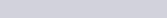; the product B is:-(1) $\mathrm{CH}_{3}-\mathrm{CH}=\mathrm{CH}-\mathrm{CHO}$(2) $\mathrm{CH}_{3}-\mathrm{CH}_{2}-\mathrm{CH}_{2}-\mathrm{CH}_{3}$(3) $\mathrm{CH}_{3}-\mathrm{CH}_{2}-\mathrm{CH}_{2}-\mathrm{CH}_{2}-\mathrm{OH}$(4)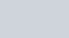[JEE(Main)-2015]

Download eSaral App for Video Lectures, Complete Revision, Study Material and much more...

Sol. (1)Q. Which compound would give 5-keto-2-methyl hexanal upon ozonlysis?[JEE(Main) 2015]

Download eSaral App for Video Lectures, Complete Revision, Study Material and much more...

Sol. (4)Biomolecule – JEE Main Previous Year Questions with Solutions
JEE Main Previous Year Papers Questions of Chemistry with Solutions are available at eSaral. Practicing JEE Mains chapter wise questions of Chemistry will help the JEE aspirants in realizing the question pattern as well as help in analyzing weak & strong areas.Simulator Previous Years AIEEE/JEE Main Questions
Q. The two functional groups present in a typical carbohydrate are :-(1) >C = O and –OH (2) –OH and –CHO (3) –OH and –COOH (4) –CHO and –COOH AIEEE-2009

Download eSaral App for Video Lectures, Complete Revision, Study Material and much more...

Sol. (2)

Q. Biurest test is not given by :-(1) proteins (2) carbohydrates (3) polypeptides (4) urea AIEEE-2010

Download eSaral App for Video Lectures, Complete Revision, Study Material and much more...

Sol. (2)

Q. The presence or absence of hydroxy group on which carbon atom of sugar differentiates RNA and DNA?(1) 3rd (2) 4th (3) 1st (4) 2nd AIEEE-2011

Download eSaral App for Video Lectures, Complete Revision, Study Material and much more...

Sol. (4)

Q. The change in the optical rotation of freshly prepared solution of glucose is known as :-(1) tautomerism (2) racemisation (3) specific rotation (4) mutarotation AIEEE-2011

Download eSaral App for Video Lectures, Complete Revision, Study Material and much more...

Sol. (4)

Q. Which one of the following statements is correct ?(1) All amino acids except glutamic acid are optically active(2) All amino acids except lysine are optically active(3) All amino acids are optically active(4) All amino acids except glycine are optically active AIEEE-2012

Download eSaral App for Video Lectures, Complete Revision, Study Material and much more...

Sol. (4)

Q. Synthesis of each molecule of glucose in photosynthesis involves :-(1) 18 molecules of ATP(2) 10 molecules of ATP(3) 8 molecules of ATP(4) 6 molecules of ATP JEE Main -2013

Download eSaral App for Video Lectures, Complete Revision, Study Material and much more...

Sol. (1)Six rounds of the Calvin cycle are required, because one carbon atom is reduced in each round. Twelve molecules of ATP are expended. An additional six molecular of ATP are spent in regenerating ribulose-1, 5-biphosphate$6 \mathrm{CO}_{2}+18 \mathrm{ATP}+12 \mathrm{NADPH}+12 \mathrm{H}_{2} \mathrm{O} \rightarrow$$\mathrm{C}_{6} \mathrm{H}_{12} \mathrm{O}_{6}+18 \mathrm{ADP}+18 \mathrm{P}_{\mathrm{i}}+12 \mathrm{NADP}+6 \mathrm{H} \oplusReference : NCERT 11th class chapter-13 (Photosynthesis Pg. 217, 218 in higher plants.Calvin cycle where sugar is synthesised is as follows :The Calvin cycle proceeds in three stages (1) carboxylation, during which \mathrm{CO}_{2} combines with ribulose-1.5-bisphosphate (2) reduction, during which carbohydrate is formed at the expense of the photochemically made ATP and NADPH and (3) regeneration during which the \mathrm{CO}_{2} acceptor ribulose-1,5-bisphosphate is formed again so that the cylcle continues.It might help you to understand all of this if we look at what goes in and what comes out of the Calvin cycle.Q. Which one of the following bases is not present in DNA ?(1) Cytosine (2) Thymine (3) Quinoline (4) Adenine JEE-Main 2014 Download eSaral App for Video Lectures, Complete Revision, Study Material and much more... Sol. (3)All cytosine, thymine and adenine are present in DNA. Only quinoline is not present in DNA. Q. Which of the vitamins given below is water soluble?(1) Vitamin E(2) Vitamin K(3) Vitamin C(4) Vitamin D JEE-Main 2015 Download eSaral App for Video Lectures, Complete Revision, Study Material and much more... Sol. (3)Vitamine C is soluble in water due to H-Bonding & all other vitamines given in question are fat soluble. Q. Complete hydrolysis of starch gives :(1) glucose and fructose in equimolar amounts(2) glucose only(3) galactose and fructose in equimolar amounts(4) glucose and galactose in equimolar amounts JEE-Main(Online) – 2015 Download eSaral App for Video Lectures, Complete Revision, Study Material and much more... Sol. (2)Starch is polymer of Glucose Q. Accumulation of which of the following molecules in the muscles occurs as a result of vigorous exercise :-(1) Pyruvic acid(2) L-lactic acid(3) Glycogen(4) Glucose JEE-Main(Online) – 2015 Download eSaral App for Video Lectures, Complete Revision, Study Material and much more... Sol. (2) Q. Consider the following sequence for aspartic acid:The pI (isoelectric point) of aspartic acid is :(1) 1.88 (2) 2.77 (3) 3.65 (4) 5.74 JEE-Main(Online) – 2016 Download eSaral App for Video Lectures, Complete Revision, Study Material and much more... Sol. (4) Q. Observation of “Rhumann’s purple” is a confirmatory test for the presence of :(1) Starch(2) Reducing sugar(3) Cupric ion(4) Protein JEE-Main(Online) – 2017 Download eSaral App for Video Lectures, Complete Revision, Study Material and much more... Sol. (1) Q. Which of the following compounds will behave as a reducing sugar in an aqueous KOH solution ?JEE-Main 2017 Download eSaral App for Video Lectures, Complete Revision, Study Material and much more... Sol. (1)(1) Ester in presence of Aqueous KOH solution give SNAE reaction so following reaction takes placeAlcohol & Ether – JEE Main Previous Year Questions with Solutions JEE Main Previous Year Papers Questions of Chemistry with Solutions are available at eSaral. Practicing JEE Mains chapter wise questions of Chemistry will help the JEE aspirants in realizing the question pattern as well as help in analyzing weak & strong areas.SimulatorPrevious Years AIEEE/JEE Mains Questions Q. The number of stereoisomers possible for a compound of the molecular formula(1) 4 (2) 6 (3) 3 (4) 2 AIEEE-2009 Download eSaral App for Video Lectures, Complete Revision, Study Material and much more... Sol. (1) Q. A liquid was mixed with ethanol and a drop of concentrated \mathrm{H}_{2} \mathrm{SO}_{4} was added. A compound with a fruity smell was formed. The liquid was :-(1) \mathrm{CH}_{3} \mathrm{COCH}_{3}(2) \mathrm{CH}_{3} \mathrm{COOH}(3) \mathrm{CH}_{3} \mathrm{OH}(4) HCHO AIEEE-2009 Download eSaral App for Video Lectures, Complete Revision, Study Material and much more... Sol. (2) Q. From amongst the following alcohols the one that would react fastest with conc. HCl and anhydrous \mathrm{ZnCl}_{2}, is :-(1) 1–Butanol(2) 2–Butanol(3) 2–Methylpropan–2–ol(4) 2–Methylpropanol AIEEE-2010 Download eSaral App for Video Lectures, Complete Revision, Study Material and much more... Sol. (3) Q. Consider the following reaction :\mathrm{C}_{2} \mathrm{H}_{5} \mathrm{OH}+\mathrm{H}_{2} \mathrm{SO}_{4} \rightarrow ProduceAmong the following, which one cannot be formed as a product under any conditions ?(1) Ethyl-hydrogen sulphate(2) Ethylene(3) Acetylene(3) Diethyl ethe AIEEE-2011 Download eSaral App for Video Lectures, Complete Revision, Study Material and much more... Sol. (3) Q. Iodoform can be prepared from all except :-(1) Isobutyl alcohol(2) Ethyl methyl ketone(3) Isopropyl alcohol(4) 3-Methyl–2–butanone AIEEE-2012 Download eSaral App for Video Lectures, Complete Revision, Study Material and much more... Sol. (1) Q. An unknown alcohol is treated with the “Lucas reagent’ to determine whether the alcohol is primary, secondary or tertiary. Which alcohol reacts fastest and by what mechanism :-(1) secondary alcoholby SN ^{1}(2) tertiary alcohol by SN ^{1}(3) secondary alcoholby SN ^{2}(4) tertiary alcohol by SN ^{2} AIEEE-2013 Download eSaral App for Video Lectures, Complete Revision, Study Material and much more... Sol. (2) Q. The most suitable reagent for the conversion of \mathrm{R}-\mathrm{CH}_{2}-\mathrm{OH} \rightarrow \mathrm{R}-\mathrm{CHO} is :(1) \mathrm{CiO}_{3}(2) PCC (Pyridinium chlorochromate)(3) KMNO _{4}(4) \mathrm{K}_{2} \mathrm{Cr}_{2} \mathrm{O}_{7} Jee-Main-2014 Download eSaral App for Video Lectures, Complete Revision, Study Material and much more... Sol. (2)Q. Allyl phenyl ether can be prepared by heating:(1) \mathrm{CH}_{2}=\mathrm{CH}-\mathrm{CH}_{2}-\mathrm{Br}+\mathrm{C}_{6} \mathrm{H}_{5} \mathrm{ONa}(2) \mathrm{C}_{6} \mathrm{H}_{5}-\mathrm{CH}=\mathrm{CH}-\mathrm{Br}+\mathrm{CH}_{3}-\mathrm{ONa}(3) \mathrm{C}_{6} \mathrm{H}_{5} \mathrm{Br}+\mathrm{CH}_{2}=\mathrm{CH}-\mathrm{CH}_{2}-\mathrm{ONa}(4) \mathrm{CH}_{2}=\mathrm{CH}-\mathrm{Br}+\mathrm{C}_{6} \mathrm{H}_{5}-\mathrm{CH}_{2}-\mathrm{ONa} Jee-Main-2014 Download eSaral App for Video Lectures, Complete Revision, Study Material and much more... Sol. (1)Q. In the Victor-Meyer’s test, the colour given by 1^{\circ}, 2^{\circ} and 3^{\circ} alcohols are respectively :-(1) Red, blue, colourless(2) Colourless, red, blue(3) Red, blue, violet(4) Red, colourless, blue Jee-Main-2014 Download eSaral App for Video Lectures, Complete Revision, Study Material and much more... Sol. (1)Q. Williamson synthesis of ether is an example of(1) Nucleophilic addition(2) Electrophilic substitution(3) Nucleophilic substitution(4) Electrophilic addition Jee-Main-2014 Download eSaral App for Video Lectures, Complete Revision, Study Material and much more... Sol. (3)Nucleophilic substitutionQ. Phenol on treatment with \mathrm{CO}_{2} in the presence of NaOH followed by acidification produces compound X as the major product. X on treatment with \left(\mathrm{CH}_{3} \mathrm{CO}\right)_{2} \mathrm{O} in the presence of catalytic amount of \mathrm{H}_{2} \mathrm{SO}_{4} produces :Jee-Main-2018 Download eSaral App for Video Lectures, Complete Revision, Study Material and much more... Sol. (4)Q. Phenol reacts with methyl chloroformate in the presence of NaOH to form product A. A reacts with \mathrm{Br}_{2} to form product B. A and B are respectively :Jee-Main-2018 Download eSaral App for Video Lectures, Complete Revision, Study Material and much more... Sol. (2)Salt Analysis – JEE Main Previous Year Questions with Solutions JEE Main Previous Year Papers Questions of Chemistry with Solutions are available at eSaral. Practicing JEE Main chapter wise questions of Chemistry will help the JEE aspirants in realising the question pattern as well as help in analysing weak & strong areas. Simulator Previous Years AIEEE/JEE Mains Questions Q. The products obtained on heating LiNO3 will be :-(1) \mathrm{LiNO}_{2}+\mathrm{O}_{2}(2) \mathrm{Li}_{2} \mathrm{O}+\mathrm{NO}_{2}+\mathrm{O}_{2}(3) \mathrm{Li}_{3} \mathrm{N}+\mathrm{O}_{2}(4) \mathrm{Li}_{2} \mathrm{O}+\mathrm{NO}+\mathrm{O}_{2} AIEEE-2011 Download eSaral App for Video Lectures, Complete Revision, Study Material and much more... Sol. (2)\mathrm{LiNO}_{3} \longrightarrow \mathrm{Li}_{2} \mathrm{O}+\mathrm{NO}_{2}+\mathrm{O} Q. What is the best description of the change that occurs when \mathrm{Na}_{2} \mathrm{O}(\mathrm{s}) is dissolved in water ?(1) Oxidation number of sodium decreases(2) Oxide ion accepts sharing in a pair of electrons(3) Oxide ion donates a pair of electrons(4) Oxidation number of oxygen increases AIEEE-2011 Download eSaral App for Video Lectures, Complete Revision, Study Material and much more... Sol. (3)\mathrm{Na}_{2} \mathrm{O}+\mathrm{HOH} \longrightarrow \mathrm{CaO}+\mathrm{CO}_{2} Q. Which of the following on thermal-decomposition yields a basic as well as an acidi oxide ?(1) \mathrm{NH}_{4} \mathrm{NO}_{3}(2) NaNO _{3}(3) \mathrm{KClO}_{3}(4) \mathrm{CaCO}_{3} AIEEE-2012 Download eSaral App for Video Lectures, Complete Revision, Study Material and much more... Sol. (4)\mathrm{CaCO}_{3} \longrightarrow \mathrm{CaO}+\mathrm{CO}_{2} Q. Fire extinguishers contain H2SO4 and which one of the following :-(1) \mathrm{CaCO}_{3}(2) \mathrm{NaHCO}_{3} and \mathrm{Na}_{2} \mathrm{CO}_{3}(3) \mathrm{Na}_{2} \mathrm{CO}_{3}(4) \mathrm{NaHCO}_{3} JEE(Main)-2012 online-P-1 Download eSaral App for Video Lectures, Complete Revision, Study Material and much more... Sol. (4)Fire extinguishers contain H2SO4 and NaHCO3 Q. Copper wire test for halogens is known as :-(1) Duma’s Test(2) Beilstein’s Test(3) Lasssigne’s Test(4) Liebig’s Test JEE(Main)-2012 online-P-2 Download eSaral App for Video Lectures, Complete Revision, Study Material and much more... Sol. (2)Beilstein’s Test Q. The standard potentials of \mathrm{Ag}^{+} / \mathrm{Ag}, \mathrm{Hg}_{2}^{2+} / 2 \mathrm{Hg}, \mathrm{Cu}^{2+} / \mathrm{Cu} and \mathrm{Mg}^{2+} / Mg electrodes are 0.80, 0.79, 0.34 and – 2.37 V, respectively. An aqueous solution which contains one mole per litre of the salts of each of the four metals is electrolyzed. With increasing voltage, the correct sequence of deposition of the metals at the cathode is :-(1) Cu, Hg, Ag only(2) Mg, Cu, Hg, Ag(3) Ag, Hg, Cu only(4) Ag, Hg, Cu, Mg [JEE(Main)-2012 online-P-3] Download eSaral App for Video Lectures, Complete Revision, Study Material and much more... Sol. (3) Q. Beilstein test is used for estimation of which one of following elements ?(1) S(2) Cl(3) C and H(4) N JEE(Main)-2012 online-P-3 Download eSaral App for Video Lectures, Complete Revision, Study Material and much more... Sol. (2)5 \mathrm{Mn} \mathrm{O}_{4}^{-}+2 \mathrm{C}_{2} \mathrm{O}_{4}^{2-}+16 \mathrm{H}^{+} \longrightarrow 5 \mathrm{Mn}^{+2}+2 \mathrm{CO}_{2}+\frac{16}{2} \mathrm{H}_{2} \mathrm{O} Q. In the following balanced reaction, \mathrm{XMnO}_{+}^{-}+\mathrm{YC}_{2} \mathrm{O}_{4}^{2-}+\mathrm{ZH}^{+}=\mathrm{XMn}^{2+}+2 \mathrm{YCO}_{2}+ \frac{\mathrm{Z}}{2} \mathrm{H}_{2} \mathrm{O} the values of X, Y and Z, respectively are :- JEE(Main)-2012 online-P-4 Download eSaral App for Video Lectures, Complete Revision, Study Material and much more... Sol. (2) Q. A metal M on heating in nitrogen gas gives Y. Y on treatment with \mathrm{H}_{2} \mathrm{O} gives a colourless gas which when passed through \mathrm{CuSO}_{4} solution gives a blue colour, Y is :-(1) \mathrm{NH}_{3}(2) MgO(3) \mathrm{Mg}_{3} \mathrm{N}_{2}(4) \mathrm{Mg}\left(\mathrm{NO}_{3}\right)_{2} JEE(Main)-2012 online-P-4 Download eSaral App for Video Lectures, Complete Revision, Study Material and much more... Sol. (3)Q. Electrode potentials (E0) are given below :\mathrm{Cu}^{+} / \mathrm{Cu}=+0.52 \mathrm{V}, \mathrm{Fe}^{3+} / \mathrm{Fe}^{2+}=+0.77 \mathrm{V}$$1 / 2 \mathrm{I}_{2}(\mathrm{s}) / \mathrm{I}^{-}=+0.54 \mathrm{V}, \mathrm{Ag}^{+} / \mathrm{Ag}=+0.88 \mathrm{V}$Based on the above potentials, strnogest oxidizing agent will be :(1) $\mathrm{Cu}^{+}$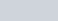(3) $\mathrm{Ag}^{+}$(4) $\mathrm{I}_{2}$ JEE(Main)-2013 online-P-1

Download eSaral App for Video Lectures, Complete Revision, Study Material and much more...

Sol. (3)

Q. Potassium dichromate when heated with concentrated sulphuric acid and a soluble chloride, gives brown – red vapours of:( 1) $\mathrm{CrO}_{3}$(2) $\mathrm{Cr}_{2} \mathrm{O}_{3}$(3) $\mathrm{CrCl}_{3}$(4) $\mathrm{CrO}_{2} \mathrm{Cl}_{2}$ JEE(Main)-2013 online-P-1

Download eSaral App for Video Lectures, Complete Revision, Study Material and much more...

Sol. (4)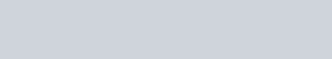Q. Given :$\overline{\mathrm{X}} \mathrm{Na}_{2} \mathrm{HAsO}_{3}+\mathrm{Y} \mathrm{NaBrO}_{3}+\mathrm{ZHCl} \rightarrow \mathrm{NaBr}+\mathrm{H}_{3} \mathrm{AsO}_{4}+\mathrm{NaCl}$The values of X, Y and Z in the above redox reaction are respectively :(1) 2, 1, 3 (2) 3, 1, 6 (3) 2, 1, 2 (4) 3, 1, 4 JEE(Main)-2013 online-P-1

Download eSaral App for Video Lectures, Complete Revision, Study Material and much more...

Sol. (2)$3 \mathrm{Na}_{2} \mathrm{HAsO}_{3}+\mathrm{NaBrO}_{3}+6 \mathrm{HCl} \longrightarrow \mathrm{NaBr}+\mathrm{H}_{3} \mathrm{AsO}_{4}+\mathrm{NaCl}$

Q. Sodium Carbonate cannot be used in place of $\left(\mathrm{NH}_{4}\right)_{2} \mathrm{CO}_{3}$ for the identification of $\mathrm{Ca}^{2+}, \mathrm{Ba}^{2+}$ and $\mathrm{Sr}^{2+}$ ions (in group V) during mixture analysis because :(1) Sodium ions will react with acid radicals(2) Concentration of $\mathrm{CO}_{3}^{2-}$ ions is very low(3) Mg $^{2+}$ ions will also be precipitated(4) $\mathrm{Na}^{+}$ ions will interfere with the detection of $\mathrm{Ca}^{2+}, \mathrm{Ba}^{2+}, \mathrm{Sr}^{2+}$ ions JEE(Main)-2013 online-P-1

Download eSaral App for Video Lectures, Complete Revision, Study Material and much more...

Sol. (3)According to reactivity series Mg can be displaced by Na.

Q. Which of the following statements is incorrect?(1) $\mathrm{Fe}^{2+}$ ion also gives blood red colour with $\mathrm{SCN}^{-}$ ion(2) Cupric ion reacts with excess of ammonia solution to give deep blue colour of $\left[\mathrm{Cu}\left(\mathrm{NH}_{3}\right)_{4}\right]^{2+}$ ion.(3) $\mathrm{Fe}^{3+}$ ion gives blood red colour with $\mathrm{SCN}^{-}$ ion.(4) On passing $\mathrm{H}_{2} \mathrm{S}$ into $\mathrm{Na}_{2} \mathrm{ZnO}_{2}$ solution, a white ppt of ZnS is formed.

Download eSaral App for Video Lectures, Complete Revision, Study Material and much more...

Sol. (1)$\mathrm{Fe}^{+2}+\mathrm{SCN}^{-} \longrightarrow$ No colour

Q. Values of dissociation constant, $\mathrm{K}_{\mathrm{a}}$ are given as follows :(1) $\mathrm{NO}_{2}^{-}<\mathrm{CN}^{-}<\mathrm{F}^{-}$(2) $\mathrm{F}^{-}<\mathrm{CN}^{-}<\mathrm{NO}_{2}^{-}$(3) $\mathrm{NO}_{2}^{-}<\mathrm{F}^{-}<\mathrm{CN}^{-}$(4) $\mathrm{F}^{-}<\mathrm{NO}_{2}^{-}<\mathrm{CN}^{-}$ JEE(Main)-2013 online-P-2

Download eSaral App for Video Lectures, Complete Revision, Study Material and much more...

Sol. (4)Order of basic strength according to Ka value.$\mathrm{CN}^{-}>\mathrm{NO}_{2}^{-}>\mathrm{F}^{-}$

Q. Identify incorrect statement(1) Copper (I) compounds are colourless except where colour results from charge transfer(2) Copper (I) compounds are diamagnetic(3) $\mathrm{Cu}_{2} \mathrm{S}$ is black(4) $\mathrm{Cu}_{2} \mathrm{O}$ is colourless JEE(Main)-2013 online-P-3

Download eSaral App for Video Lectures, Complete Revision, Study Material and much more...

Sol. (4)$\mathrm{Cu}_{2} \mathrm{O}$ is red.

Q. Given :$\mathrm{E}_{1 / 2 \mathrm{C}_{2} / \mathrm{Cl}^{-}}^{0}=1.36 \mathrm{V}, \mathrm{E}_{\mathrm{Cr}^{3+} / \mathrm{Gr}}^{0}=-0.74 \mathrm{V} ; \quad \mathrm{E}_{\mathrm{G}_{2} \mathrm{O}_{7}^{2-} / \mathrm{Cr}^{3+}}^{0}=1.33 \mathrm{V}, \mathrm{E}_{\mathrm{MnO}_{4}^{-} / \mathrm{Mn}^{2+}}^{0}=1.51 \mathrm{V}$The correct order of reducing power of the species $\left(\mathrm{Cr}, \mathrm{Cr}^{3+}, \mathrm{Mn}^{2+} \text { and } \mathrm{Cl}^{-}\right)$ will be : JEE(Main)-2013 online-P-3(1) $\mathrm{Mn}^{2+}<\mathrm{Cl}^{-}<\mathrm{Cr}^{3+}<\mathrm{Cr}$(2) $\mathrm{Cr}^{3+}<\mathrm{Cl}^{-}<\mathrm{Mn}^{2+}<\mathrm{Cr}$(3) $\mathrm{Cr}^{3+}<\mathrm{Cl}^{-}<\mathrm{Cr}<\mathrm{Mn}^{2+}$(4) $\mathrm{Mn}^{2+}<\mathrm{Cr}^{3+}<\mathrm{Cl}^{-}<$

Download eSaral App for Video Lectures, Complete Revision, Study Material and much more...

Sol. (1)Order of reducing power acoording to E$\mathrm{Cr}>\mathrm{Cr}^{+3}>\mathrm{Cl}^{-}>\mathrm{Mn}^{+2}$

Q. Which one of the following cannot function as an oxidising agent ?(1) $\mathrm{NO}_{3}^{-}(\mathrm{aq})$( 2) $\mathrm{I}^{-}$(3) $\mathrm{Cr}_{2} \mathrm{O}_{7}^{2-}$( 4) $\mathrm{S}_{(\mathrm{S})}$ JEE(Main)-2014 online_P-2

Download eSaral App for Video Lectures, Complete Revision, Study Material and much more...

Sol. (2)$\mathrm{I}^{-}$ has lowest oxidation state.

Q. Which of the following statements about $\mathrm{Na}_{2} \mathrm{O}_{2}$ is not correct ?(1) $\mathrm{Na}_{2} \mathrm{O}_{2}$ oxidises $\mathrm{Cr}^{3+}$ to $\mathrm{CrO}_{4}^{2-}$ in acid medium(2) It is diamagnetic in nature(3) It is the super oxide of sodium(4) It is a derivative of $\mathrm{H}_{2} \mathrm{O}_{2}$ JEE(Main)-2014 online_P-2

Download eSaral App for Video Lectures, Complete Revision, Study Material and much more...

Sol. (2)$\mathrm{Na}_{2} \mathrm{O}_{2}$ is peroxide

Q. Given :-$\mathrm{Fe}^{3+}(\mathrm{aq})+\mathrm{e}^{-} \rightarrow \mathrm{Fe}^{2+}(\mathrm{aq}) ; \mathrm{E}^{\circ}=+0.77 \mathrm{V}$$\mathrm{A}^{3+}(\mathrm{aq})+3 \mathrm{e}^{-} \rightarrow \mathrm{Al}(\mathrm{s}) ; \mathrm{E}^{\circ}=-1.66 \mathrm{V}$$\mathrm{Br}_{2}(\mathrm{aq})+2 \mathrm{e}^{-} \rightarrow 2 \mathrm{Br}^{-} ; \mathrm{E}^{\circ}=+1.09 \mathrm{V}$Considering the electrode potentials, which of the following represents the correct order of reducing power?(1) $\mathrm{Al}<\mathrm{Fe}^{2+}<\mathrm{Br}^{-}$(2) $\mathrm{Al}<\mathrm{Br}^{-}<\mathrm{Fe}^{2+}$(3) $\mathrm{Fe}^{2+}<\mathrm{Al}<\mathrm{Br}^{-}$$(4) \mathrm{Br}^{-}<\mathrm{Fe}^{2+}<\mathrm{Al} JEE(Main)-2014 online-P-2 Download eSaral App for Video Lectures, Complete Revision, Study Material and much more... Sol. (4)Order of reducing power according to \mathrm{E}^{\circ} \mathrm{Br}^{-}<\mathrm{Fe}^{+2}<\mathrm{Al} Q. Consider the following equilibrium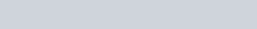White precipitate of AgCl appears on adding which of the following?(1) \mathrm{NH}_{3}(2) Aqueous NaCl(3) Aqueous \mathrm{NH}_{4} \mathrm{Cl}(4) AqueousHNO _{3} JEE(Main)-2014 online-P-2 Download eSaral App for Video Lectures, Complete Revision, Study Material and much more... Sol. (4)\left[\mathrm{Ag}\left(\mathrm{NH}_{3}\right)_{4}\right] \mathrm{Cl}+\mathrm{aqH} \mathrm{NO}_{3} \longrightarrow \mathrm{AgCl} \downarrow Q. Copper becomes green when exposed to moist air for a long period. This is due to :-(1) the formation of a layer of cupric oxide on the surface of copper.(2) the formation of basic copper sulphate layer on the surface of the metal(3) the formation of a layer of cupric hydroxide on the surface of copper.(4) the formation of a layer of basic carbonate of copper on the surface of copper. JEE(Main)-2014 online-P-3 Download eSaral App for Video Lectures, Complete Revision, Study Material and much more... Sol. (4)\mathrm{Cu} \frac{\text { Moist }}{\mathrm{air}}>\mathrm{CuCO}_{3} \cdot \mathrm{Cu}(\mathrm{OH})_{2} Q. The correct statement for the molecule, \mathrm{CsI}_{3}, is:(1) it contains \mathrm{Cs}^{3+} and \mathrm{I}^{-} ions(2) dit contains \mathrm{Cs}^{3+} and \mathrm{I}^{-} ions(3) it is a covalent molecule(4) it contains \mathrm{Cs}^{+} and \mathrm{I}_{3}^{-} ions JEE(Main)-2014 Download eSaral App for Video Lectures, Complete Revision, Study Material and much more... Sol. (4)\mathrm{CsI}_{3} \longrightarrow \mathrm{Cs}^{+}+\mathrm{I}_{3}^{-} Q. Which one of the following exhibits the largest number of oxidation states ?(1) Mn(25)(2) V(23)(3) Cr (24)(4) Ti (22) JEE(Main)-2014 online-P-3 Download eSaral App for Video Lectures, Complete Revision, Study Material and much more... Sol. (1)Mn has maximum number of oxidation state. Q. Hydrogen peroxide acts both as an oxidising and as a reducing agent depending upon the nature of the reacting species. In which of the following cases \mathrm{H}_{2} \mathrm{O}_{2} acts as a reducing agent in acid medium ? :- JEE(Main)-2014 online-P-3(1) \mathrm{MnO}_{4}^{-}(2) \mathrm{SO}_{3}^{2-}(3) KI(4) \mathrm{Cr}_{2} \mathrm{O}_{7}^{2-} Download eSaral App for Video Lectures, Complete Revision, Study Material and much more... Sol. (1)\mathrm{H}_{2} \mathrm{O}_{2} acts as a reducing agent in acidic medium in pressure of \mathrm{MnO}_{4}^{-} Q. Consider the reaction\mathrm{H}_{2} \mathrm{SO}_{3(\mathrm{aq})}+\mathrm{Sn}_{(\mathrm{aq})}^{4+}+\mathrm{H}_{2} \mathrm{O}_{(\mathrm{l})} \rightarrow \mathrm{Sn}_{(\mathrm{aq})}^{2+}+\mathrm{HSO}_{4(\mathrm{aq})}^{-}+3 \mathrm{H}_{(\mathrm{aq})}^{+}Which of the following statements is correct?(1) \mathrm{H}_{2} \mathrm{SO}_{3} is the reducing agent because it undergoes oxidation(2) \mathrm{H}_{2} \mathrm{SO}_{3} is the reducing agent because it undergoes reduction(3) \mathrm{Sn}^{4+} is the reducing agent because it undergoes oxidation(4) \mathrm{Sn}^{4+} is the oxidizing agent because it undergoes oxidation JEE(Main)-2014 online-P-4 Download eSaral App for Video Lectures, Complete Revision, Study Material and much more... Sol. (1) Q. How many electrons are involved in the following redox reaction ?\mathrm{Cr}_{2} \mathrm{O}_{7}^{2-}+\mathrm{Fe}^{2+}+\mathrm{C}_{2} \mathrm{O}_{4}^{2-} \rightarrow \mathrm{Cr}^{3+}+\mathrm{Fe}^{3+}+\mathrm{CO}_{2} (Unbalanced)(1) 3 (2) 4 (3) 5 (4) 6 JEE(Main)-2014 online-P-4 Download eSaral App for Video Lectures, Complete Revision, Study Material and much more... Sol. (4)6 e^{-} are involved in this reaction Q. Amongst the following, identify the species with an atom in +6 oxidation state:(1) \left[\mathrm{MnO}_{4}\right]^{-}(2) \left[\mathrm{Cr}(\mathrm{CN})_{6}\right]^{3-}(3) \mathrm{Cr}_{2} \mathrm{O}_{3}(4) \mathrm{Cr} \mathrm{o}_{2} \mathrm{Cl}_{2} JEE(Main)-2014 online-P-4 Download eSaral App for Video Lectures, Complete Revision, Study Material and much more... Sol. (4)\mathrm{CrO}_{2} \mathrm{Cl}_{2} \mathrm{x}+(-4)+(-2)=0$$\mathrm{x}=+6$

Q. Which of the following salts is the most basic in aqueous solution ?( 1) $\mathrm{CH}_{3} \mathrm{COOK}$( 2) $\mathrm{FeCl}_{3}$(3) $\mathrm{Pb}\left(\mathrm{CH}_{3} \mathrm{COO}\right)_{2}$(4) $\mathrm{Al}(\mathrm{CN})_{3}$ JEE(Main)-2018

Download eSaral App for Video Lectures, Complete Revision, Study Material and much more...

Sol. (1)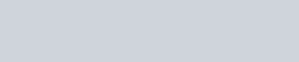Surface Chemistry – JEE Main Previous Year Questions with Solutions
JEE Main Previous Year Papers Questions of Chemistry with Solutions are available at eSaral. Practicing JEE Main chapter wise questions of Chemistry will help the JEE aspirants in realizing the question pattern as well as help in analyzing weak & strong areas. Simulator Previous Years AIEEE/JEE Main Questions
Q. Which of the following statements is incorrect regarding physisorptions ?(1) Under high pressure it results into multi molecular layer on adsorbent surface(2) Enthalpy of adsorption $\left(\Delta \mathrm{H}_{\text {adsorption }}\right)$ is low andpositive(3) It occurs because of Van der Waal’s forces(4) More easily liquefiable gases are adsorbed readi AIEEE-2009

Download eSaral App for Video Lectures, Complete Revision, Study Material and much more...

Sol. (2)$\Delta \mathrm{H}$ is negative

Q. According to Freundlich adsorption isotherm, which of the following is correct ?(1) $\frac{\mathrm{x}}{\mathrm{m}} \propto \mathrm{p}^{0}$(2) $\frac{\mathrm{x}}{\mathrm{m}} \propto \mathrm{p}^{1}$(3) $\frac{\mathrm{x}}{\mathrm{m}} \propto \mathrm{p}^{1 / \mathrm{n}}$(4) All the above are correct for different ranges of pressure AIEEE-2012

Download eSaral App for Video Lectures, Complete Revision, Study Material and much more...

Sol. (4)Q. The coagulating power of electrolytes having ions $\mathrm{Na}^{+}, \mathrm{Al}^{3+}$ and $\mathrm{Ba}^{2+}$ for aresenic sulphide sol increases in the order :-(1) $\mathrm{Al}^{3+}<\mathrm{Ba}^{2+}<\mathrm{Na}^{+}$(2) $\mathrm{Na}^{+}<\mathrm{Ba}^{2+}<\mathrm{Al}^{3+}$.(3) $\mathrm{Ba}^{2+}<\mathrm{Na}^{+}<\mathrm{Al}^{3+}$(4) $\mathrm{Al}^{3+}<\mathrm{Na}^{+}<\mathrm{Ba}^{2+}$ JEE-Main 2013

Download eSaral App for Video Lectures, Complete Revision, Study Material and much more...

Sol. (2)According to hardley schuzle rule

Q. For a linear plot of log(x/m) versus log p in a Freundlich adsorption isotherm, which of the following statements is correct ? (k and n are constants)(1) log (1/n) appears as the intercept(2) Both k and 1/n appear in the slope term(3) 1/n appears as the intercept(4) Only 1/n appears as the slope JEE-Main 2016

Download eSaral App for Video Lectures, Complete Revision, Study Material and much more...

Sol. (4)According to Freundlich isothermQ. The Tyndall effect is observed only when following conditions are satisfied :-(a) The diameter of the dispersed particles is much smaller than the wavelength of the lighused.(b) The diameter of the dispersed particle is not much smaller than the wavelength of the lightused.(c) The refractive indices of the dispersed phase and dispersion medium are almost similar inmagnitude.(d) The refractive indices of the dispersed phase and dispersion medium differ greatly inmagnitude.(1) (a) and (d)(2) (b) and (d)(3) (a) and (c)(4) (b) and (c) JEE – Main – 2017

Download eSaral App for Video Lectures, Complete Revision, Study Material and much more...

Sol. (2)As per NCERT book (fact)

Solid State – JEE Main Previous Year Questions with Solutions
JEE Main Previous Year Papers Questions of Chemistry with Solutions are available at eSaral. Practicing JEE Main chapter wise questions of Chemistry will help the JEE aspirants in realizing the question pattern as well as help in analyzing weak & strong areas. SimulatorPrevious Years AIEEE/JEE Mains Questions
Q. The edge length of a face centered cubic cell of an ionic substance is 508 pm. If the radius of the cation is 110 pm, the radius of the anion is :-(1) 144 pm (2) 288 pm (3) 398 pm (4) 618 pm Aieee-10

Download eSaral App for Video Lectures, Complete Revision, Study Material and much more...

Sol. (1)radius of cation = 110 pmif cation present 2n OV$a=2\left(r_{+}+r_{-}\right)$508 = 2[110 + x]x = 144 pm

Q. Percentages of free space in cubic close packed structure and in body centered packed structure are respectively :-(1) 48% and 26% (2) 30% and 26% (3) 26% and 32% (4) 32% and 48% Aieee-10

Download eSaral App for Video Lectures, Complete Revision, Study Material and much more...

Sol. (3)Theory Based

Q. Copper crystallises in fcc lattice with a unit cell edge of 361 pm. The radius of copper atom is:-(1) 181 pm (2) 108 pm (3) 128 pm (4) 157 pm Aieee-11 / JEE-Main-ONLINE 2013

Download eSaral App for Video Lectures, Complete Revision, Study Material and much more...

Sol. (3)

Q. In a face centred cubic lattice, atom A occupies the corner positions and atom B occupies the face centre positions. If one atom of B is missing from one of the face centred points, the formula of the compound is :-(1) $\mathrm{A}_{2} \mathrm{B}_{3}$( 2) $\mathrm{A}_{2} \mathrm{B}_{5}$(3) $\mathrm{A}_{2} \mathrm{B}$(4) $\mathrm{AB}_{2}$ Aieee-11 / JEE-Main-ONLINE 2014

Download eSaral App for Video Lectures, Complete Revision, Study Material and much more...

Sol. (2)

Q. Lithium forms body centred cubic structure. The length of the side of its unit cell is 351 pm. Atomic radius of the lithium will be :- (1) 152 pm (2) 75 pm (3) 300 pm (4) 240 pm Aieee-12

Download eSaral App for Video Lectures, Complete Revision, Study Material and much more...

Sol. (1)Q. The radius of a calcium ion is 94 pm and of the oxide ion is 146 pm. The possible crystal structure of calcium oxide will be :-(1) Octahedral        (2) Tetrahedral          (3) Pyramidal           (4) Trigonal Jee-Main (online)-12

Download eSaral App for Video Lectures, Complete Revision, Study Material and much more...

Sol. (1)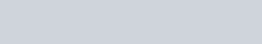Q. Ammonium chloride crystallizes in a body centred cubic lattice with edge length of unit cell of 390 pm. If the size of chloride ion is180 pm, the size of ammonium ion would be:(1) 158 pm (2) 174 pm (3) 142 pm (4) 126 pm Jee-Main (online)-12

Download eSaral App for Video Lectures, Complete Revision, Study Material and much more...

Sol. (1)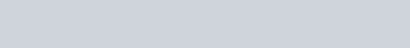Q. A solid has ‘bcc’ structure. If the distance of nearest approach between two atoms is 1.73 Å, the edge length of the cell is :-(1) 314.20 pm (2) 216 pm (3) 200 pm (4) 1.41 pm Jee-Main (online)-12

Download eSaral App for Video Lectures, Complete Revision, Study Material and much more...

Sol. (3)Q. Among the following the incorrect statement is :- (1) Density of crystals remains unaffecfted due to Frenkel defect(2) In BCC unit cell the void space is 32%(3) Electrical conductivity of semiconductors and metals increases with increase in temperature(4) Density of crystals decreases due to Schottky defect Jee-Main (online)-12

Download eSaral App for Video Lectures, Complete Revision, Study Material and much more...

Sol. (3)Theory Based

Q. In a face centred cubic lattice, atoms of A form the corner points and atoms of B form the face centred points. If two atoms of A are missing from the corner points, the formula of the ionic compound is(1) $\mathrm{AB}_{2}$( 2) $\mathrm{AB}_{3}$(3) $\mathrm{AB}_{4}$(4) $\mathrm{A}_{2} \mathrm{B}_{5}$ Jee-Main (online)-13

Download eSaral App for Video Lectures, Complete Revision, Study Material and much more...

Sol. (3)Q. Which one of the following statements about packing in solids is incorrect ?(1) Void space in ccp mode of packing is 26%(2) Coordination number in hcp mode of packing is 12(3) Void space in hcp mode of packing is 32%(4) Coordination number in bcc mode of packing is 8 Jee-Main (online)-13

Download eSaral App for Video Lectures, Complete Revision, Study Material and much more...

Sol. (3)Theory based

Q. An element having an atomic radius of 0.14 nm crystallizes in an fcc unit cell. What is the length of a side of the cell ?(1) 0.96 nm (2) 0.4 nm (3) 0.24 nm (4) 0.56 nm Jee-Main (online)-13

Download eSaral App for Video Lectures, Complete Revision, Study Material and much more...

Sol. (2)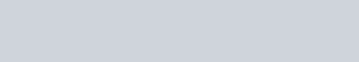Q. Expermentally it was found that a metal oxide has formula $\mathrm{M}_{0.98} \mathrm{O}$. Metal M, is present as $\mathrm{M}^{2+}$ and $\mathbf{M}^{3+}$ in its oxide. Fraction of the metal which exists as $\mathrm{M}^{3+}$ would be :-(1) 7.01%          (2) 4.08%           (3) 6.05%           (4) 5.08 Jee-Main (offline)-13

Download eSaral App for Video Lectures, Complete Revision, Study Material and much more...

Sol. (2)Q. The total number of octahedral void(s) per atom present in a cubic close packed structure is :-(1) 1          (2) 2              (3) 3              (4) 4 Jee-Main (online)-14

Download eSaral App for Video Lectures, Complete Revision, Study Material and much more...

Sol. (1)Theory based

Q. In a monoclinic unit cell, the relation of sides and angles are respectively(1) $\mathrm{a} \neq \mathrm{b} \neq \mathrm{c}$ and $\alpha \neq \beta \neq \gamma \neq 90^{\circ}$(2) $a \neq b \neq c$ and $\beta=\gamma=90^{\circ} \neq \alpha$(3) $a=b \neq c$ and $\alpha=\beta=\gamma=90^{\circ}$(4) $a \neq b \neq c$ and $\alpha=\beta=\gamma=90^{\circ}$ Jee-Main (online)-14

Download eSaral App for Video Lectures, Complete Revision, Study Material and much more...

Sol. (2)Theory based

Q. The appearance of colour in solid alkali metal halides is generally due to :(1) Frenkel defect (2) F-centres (3) Schottky defect (4) Interstitial position Jee-Main (online)-14

Download eSaral App for Video Lectures, Complete Revision, Study Material and much more...

Sol. (2)Theory based

Q. CsCl crystallises in body centred cubic lattice. if ‘a’ is its edge length then which of the following expression is correct :(1) $\mathrm{r}_{\mathrm{Cs}^{+}}+\mathrm{r}_{\mathrm{Cl}^{-}}=\frac{\sqrt{3}}{2} \mathrm{a}$(2) $\mathrm{r}_{\mathrm{Cs}^{+}}+\mathrm{r}_{\mathrm{Cl}^{-}}=\sqrt{3} \mathrm{a}$(3) $\quad \mathrm{r}_{\mathrm{Cs}^{+}}+\mathrm{r}_{\mathrm{Cl}^{-}}=3 \mathrm{a}$(4) $\mathrm{r}_{\mathrm{Cs}^{+}}+\mathrm{r}_{\mathrm{Cl}^{-}}=\frac{3 \mathrm{a}}{2}$ Jee-Main (offline)-14

Download eSaral App for Video Lectures, Complete Revision, Study Material and much more...

Sol. (1)Q. A metal crystallises in a face centred cubic structure. If the edge length of its unit cell is ‘a’, the closest approach between two atoms in metallic crystal will be :- (1) 2a(2) $2 \sqrt{2} \mathrm{a}$(3) $\sqrt{2} \mathrm{a}$(4) $\frac{\mathrm{a}}{\sqrt{2}}$ JEE – Main 2017

Download eSaral App for Video Lectures, Complete Revision, Study Material and much more...

Sol. (4)Q. Which type of ‘defect’ has the presence of cations in the interstitial sites ?(1) Vacancy defect(2) Frenkel defect(3) Metal deficiency defect(4) Schottky defect JEE – Main 2018

Download eSaral App for Video Lectures, Complete Revision, Study Material and much more...

Sol. (2)In Frenkel defect, some of ion (usually cation due to their small size) missing from their correct position and occupies position in interstitial.

Nuclear Chemistry – JEE Main Previous Year Questions with Solutions
JEE Main Previous Year Papers Questions of Chemistry with Solutions are available at eSaral. Practicing JEE Mains chapter wise questions of Chemistry will help the JEE aspirants in realizing the question pattern as well as help in analyzing weak & strong areas.Electrochemistry – JEE Main Previous Year Questions with Solutions
JEE Main Previous Year Papers Questions of Chemistry with Solutions are available at eSaral. Practicing JEE Main chapter wise questions of Chemistry will help the JEE aspirants in realizing the question pattern as well as help in analyzing weak & strong areas. Simulator Previous Years AIEEE/JEE Mains Questions
Q. Given :$\mathrm{E}_{\mathrm{Fe}^{-3} / \mathrm{Fe}}^{\circ}=-0.036 \mathrm{V}, \quad \mathrm{E}_{\mathrm{Fe}^{-2} / \mathrm{Fe}}^{\circ}=-0.439 \mathrm{V}$. The value of standard electrode potential for the change.$\mathrm{Fe}^{+3}_{(\mathrm{aq})}+\mathrm{e}^{-} \longrightarrow \mathrm{Fe}^{+2}_{(\mathrm{aq})}$ will be :-(1) 0.770 V (2) –0.27 V (3) –0.072 V (4) 0.385 V AIEEE 2009

Download eSaral App for Video Lectures, Complete Revision, Study Material and much more...

Sol. (1)$\mathrm{E}^{\circ}=\frac{3 \times-0.036+2 \times 0.439}{1}=0.77 \Rightarrow \mathrm{A}$

Q. The Gibbs energy for the decomposition of $\mathrm{Al}_{2} \mathrm{O}_{3}$ at $500^{\circ} \mathrm{C}$ is as follows :$\frac{2}{3} \mathrm{Al}_{2} \mathrm{O}_{3} \longrightarrow \frac{4}{3} \mathrm{Al}+\mathrm{O}_{2}, \mathrm{AG}=+966 \mathrm{KJ} \mathrm{mol}^{-1}$ The potential difference needed for electrolytic reduction of $\mathrm{Al}_{2} \mathrm{O}_{3}$ at $500^{\circ} \mathrm{C}$ is at least :-(1) 5.0 V      (2) 4.5 V       (3) 3.0 V         (4) 2.5 V AIEEE 2010

Download eSaral App for Video Lectures, Complete Revision, Study Material and much more...

Sol. (4)$966 \times 10^{3}=4 \times 96500 \times \mathrm{E} \Rightarrow \mathrm{E}=2.5 \mathrm{V}$

Q. Resistance of 0.2 M solution of an electrolyte is $50 \Omega$. The specific conductance of the solution is 1.3 S $\mathrm{m}^{-1}$. If resistance of the 0.4M solution of the same electrolyte is $260 \Omega$, its molar conductivity is :-(1) $6250 \mathrm{Sm}^{2} \mathrm{mol}^{-1}$(2) $6.25 \times 10^{-4} \mathrm{S} \mathrm{m}^{2} \mathrm{mol}^{-1}$(3) $625 \times 10^{-4} \mathrm{S} \mathrm{m}^{2} \mathrm{mol}^{-1}$(4) $62.5 \mathrm{S} \mathrm{m}^{2} \mathrm{mol}^{-1}$ AIEEE 2011/JEE-MAIN 2014

Download eSaral App for Video Lectures, Complete Revision, Study Material and much more...

Sol. (2)Q. The reduction potential of hydrogen half-cell will be negative if :-(1) $\mathrm{p}\left(\mathrm{H}_{2}\right)=2 \operatorname{atm}\left[\mathrm{H}^{+}\right]=1.0 \mathrm{M}$(2) $\mathrm{p}\left(\mathrm{H}_{2}\right)=2$ atm and $\left[\mathrm{H}^{+}\right]=2.0 \mathrm{M}$(3) $\mathrm{p}\left(\mathrm{H}_{2}\right)=1$ atm and $\left[\mathrm{H}^{+}\right]=2.0 \mathrm{M}$(4) $\mathrm{p}\left(\mathrm{H}_{2}\right)=1$ atm and $\left[\mathrm{H}^{+}\right]=1.0 \mathrm{M}$ AIEEE 2011

Download eSaral App for Video Lectures, Complete Revision, Study Material and much more...

Sol. (1)Q. The standard reduction potentials for $\mathrm{Zn}^{2+} / \mathrm{Zn}, \mathrm{Ni}^{2+} / \mathrm{Ni}$ and $\mathrm{Fe}^{2+} / \mathrm{Fe}$ are $-0.76,-0.23$ and – 0.44 V respectively. The reaction $\mathrm{X}+\mathrm{Y}^{+2} \rightarrow \mathrm{X}^{2+}+\mathrm{Y}$ will be spontaneous when(1) X = Zn, Y = Ni(2) X = Ni, Y = Fe(3) X = Ni, Y = Zn(4) X = Fe, Y = Zn

Download eSaral App for Video Lectures, Complete Revision, Study Material and much more...

Sol. (1)For spontaneous reaction $\mathrm{E}^{\circ}>0 \mathrm{so}(\mathrm{A})$

Q. Given :Based on the data given above, strongest oxidising agent will be :(1) Cl–(2) $\mathrm{Cr}^{3+}$(3) $\mathrm{Mn}^{2+}$(4) $\mathrm{MnO}_{4}^{-}$ JEE-Mains 2013

Download eSaral App for Video Lectures, Complete Revision, Study Material and much more...

Sol. (4)Higher the SRP, stronger will be oxidising agentHence, $\mathrm{MnO}_{4}^{-}$ is stronger oxidising agent.

Q. The equivalent conductance of NaCl at concentration C and at infinite dilution are $\lambda_{\mathrm{C}}$ and $\lambda_{\infty}$ , respectively. The correct relationship between $\lambda_{\mathrm{C}}$ and $\lambda_{\infty}$ is given as:(1) $\lambda_{\mathrm{C}}=\lambda_{\infty}-(\mathrm{B}) \sqrt{\mathrm{C}}$(2) $\lambda_{\mathrm{C}}=\lambda_{\infty}+(\mathrm{B}) \sqrt{\mathrm{C}}$(3) $\lambda_{\mathrm{C}}=\lambda_{\infty}+(\mathrm{B}) \mathrm{C}$(4) $\lambda_{\mathrm{C}}=\lambda_{\infty}-(\mathrm{B}) \mathrm{C}$ JEE-Mains 2014

Download eSaral App for Video Lectures, Complete Revision, Study Material and much more...

Sol. (1)Fact

Q. At 298 K, the standard reduction potentials are 1.51 V for $\mathrm{MnO}_{4}-| \mathrm{Mn}^{2+}$ , 1.36 V for $\mathrm{Cl}_{2} | \mathrm{Cl}^{-}$, 1.07 V for $\mathrm{Br}_{2} | \mathrm{Br}^{-},$ and $0.54 \mathrm{V}$ for $\mathrm{I}_{2} | \mathrm{I}^{-} .$ At $\mathrm{pH}=3$, permanganate is expected to oxidize $\left(\frac{\mathrm{RT}}{\mathrm{F}}=0.059 \mathrm{V}\right):-$(1) $\mathrm{Cl}^{-}$ and $\mathrm{Br}^{-}$(2) $\mathrm{Cl}^{-}, \mathrm{Br}^{-}$ and $\mathrm{I}^{-}$(3) $\mathrm{Br}^{-}$ and $\mathrm{I}^{-}$(4) I- only JEE-Mains (online) 2015

Download eSaral App for Video Lectures, Complete Revision, Study Material and much more...

Sol. (3)Q. A variable, opposite external potential $\left(\mathrm{E}_{\mathrm{ext}}\right)$ is applied to the cell$\mathrm{Zn}\left|\mathrm{Zn}^{2+}(1 \mathrm{M}) \| \mathrm{Cu}^{2+}(1 \mathrm{M})\right| \mathrm{Cu}$, of potential 1.1 V. When $\mathrm{E}_{\mathrm{ext}}<1.1 \mathrm{V}$ and $\mathrm{E}_{\mathrm{ext}}>1.1 \mathrm{V}$ , respectively electrons flow from :(1) anode to cathode in both cases(2) anode to cathode and cathode to anode(3) cathode to anode in both cases(4) cathode to anode and anode to cathode JEE-Mains (online) 2015

Download eSaral App for Video Lectures, Complete Revision, Study Material and much more...

Sol. (2)Fact

Q. Two Faraday of electricity is passed through a solution of $\mathrm{CuSO}_{4}$. The mass of copper deposited at the cathode is :(at. mass of Cu = 63.5 amu)(1) 2g         (2) 127 g          (3) 0 g          (4) 63.5 g JEE-Mains 2015

Download eSaral App for Video Lectures, Complete Revision, Study Material and much more...

Sol. (4)$2 \mathrm{F}=2 \mathrm{eq}=1$ mole $=63.5 \mathrm{gm}$

Q. Galvanization is applying a coating of :-(1)Zn         (2) Pb         (3) Cr        (4) Cu JEE-Mains 2016

Download eSaral App for Video Lectures, Complete Revision, Study Material and much more...

Sol. (1)Galvanization is the process of applying a protective zinc coating of steel or iron, to prevent rusting.

Q. Given(1)Cr(2) $\mathrm{Mn}^{2+}$(3) $\mathrm{Cr}^{3+}$(4) $\mathrm{Cl}^{-}$ JEE-Mains 2017

Download eSaral App for Video Lectures, Complete Revision, Study Material and much more...

Sol. (1)Since $\mathrm{Cr}^{+3}$ is having least reducing potential, so Cr is the best Reducing agent.

Q. How long (approximate) should water be electrolysed by passing through 100amperes current so that the oxygen released can completely burn 27.66 g of diborane ?(Atomic weight of B = 10.8 u)(1)0.8 hours(2) 3.2 hours(3) 1.6 hours(4) 6.4 hours JEE-Mains 2018

Download eSaral App for Video Lectures, Complete Revision, Study Material and much more...

Sol. (2)